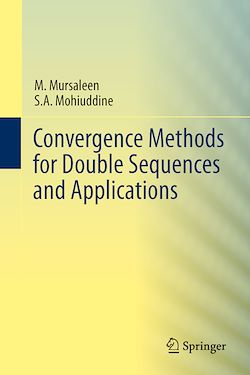# Convergence Methods for Double Sequences and Applications

-English

Description

This book exclusively deals with the study of almost convergence and statistical convergence of double sequences. The notion of “almost convergence” is perhaps the most useful notion in order to obtain a weak limit of a bounded non-convergent sequence. There is another notion of convergence known as the “statistical convergence”, introduced by H. Fast, which is an extension of the usual concept of sequential limits. This concept arises as an example of “convergence in density” which is also studied as a summability method. Even unbounded sequences can be dealt with by using this method. The book also discusses the applications of these non-matrix methods in approximation theory. Written in a self-contained style, the book discusses in detail the methods of almost convergence and statistical convergence for double sequences along with applications and suitable examples. The last chapter is devoted to the study convergence of double series and describes various convergence tests analogous to those of single sequences. In addition to applications in approximation theory, the results are expected to find application in many other areas of pure and applied mathematics such as mathematical analysis, probability, fixed point theory and statistics.

Subjects

##### Mathematical analysis

Informations